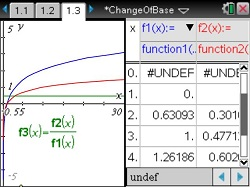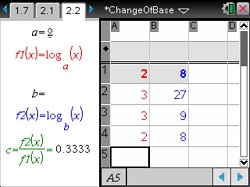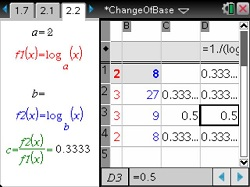# Activities

••• ##### Subject Area

• Math: Precalculus: Logarithmic Functions
• Math: College Algebra: Exponential and Logarithmic Functions

• ##### Author9-12

40 Minutes

• ##### Device
•TI-Nspire™ CX/CX II
•TI-Nspire™ CX CAS/CX II CAS
• ##### Software

TI-Nspire™
TI-Nspire™ CAS

3.0

• ##### Report an Issue

Change Of Base#### Activity Overview

Discover the change of base rule for logarithms by examining the ratio of two logarithmic functions with different bases.

#### Key Steps

•In Problem 1, students compare the graphs of two logarithmic functions with different bases. They are to use the Trace feature to determine the values of the bases.

Students then use a function table to determine the constant factor of the two functions.

•In Problem 2, students investigate the effect of the bases on the value of c by testing different values of the bases of logarithmic functions in the spreadsheet.

•Then students find a formula to calculate c algebraically by rewriting the general logarithmic function and using the properties of logs. They can then use the formula for c, to change the base of a logarithmic function.